# Reduced Drift-MHD Model

Following Section 5.2, it is convenient to set the normalization scale-length,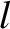, (see Section 4.3) in our reduced drift-MHD model equal to the minor radius of the rational surface,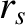. The model evolves four scalar fields. These fields, which are defined in Equations (5.1)–(5.4), are the (normalized) helical magnetic flux,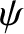, the (normalized) perturbed total plasma pressure,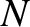, the (normalized) MHD fluid stream-function,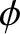, and the (normalized) ion parallel velocity,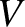. The four fields, as well as the auxiliary field,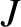, which is defined in Equation (5.8), are assumed only to be functions of the normalized radial coordinate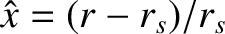, the helical angle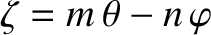, and the normalized time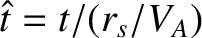. Here,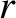,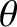,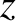are conventional cylindrical coordinates,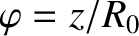a simulated toroidal angle (see Chapter 3),the simulated major radius of the plasma, and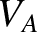the Alfvén speed [see Equation (4.23)].

It is convenient to work in a frame of reference that co-rotates with the island chain. [This goal can be achieved by making the transformation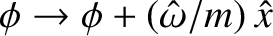. See the following paragraph for the definitions of these quantities.] In the co-rotating reference frame, the normalized reconnected flux at the rational surface,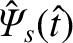[see Equation (3.184)], is assumed to be a positive real quantity. It is helpful to define the reduced (by a factor four) radial width of the magnetic island chain that develops in the inner region: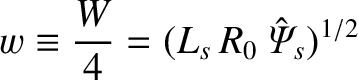(8.1)

[see Equation (5.129)]. Here,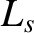is the magnetic shear-length at the rational surface [see Equation (5.27)].

In the following, it is assumed that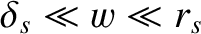, where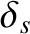is the linear layer width. (See Chapter 6.) In other words, the width of the island chain is assumed to be much greater than the linear layer width, but much less than the minor radius of the rational magnetic flux-surface. Let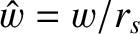. Reusing the analysis of Section 5.3, we find that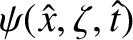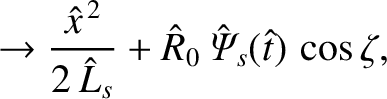(8.2)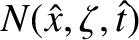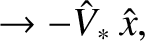(8.3)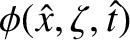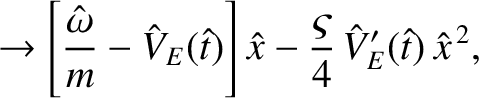(8.4)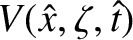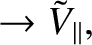(8.5)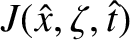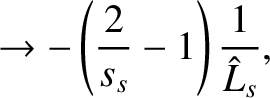(8.6)

in the limit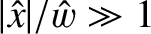(i.e., many island widths from the rational surface). Here,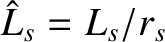,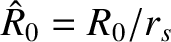,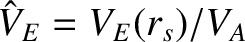,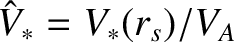,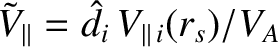,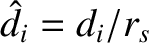, and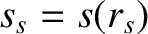, where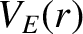is the E-cross-B velocity profile in the outer region [see Equation (5.21)],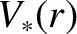the diamagnetic velocity profile [see Equation (5.29)],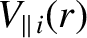the ion parallel velocity profile [see Equation (5.19)],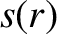the magnetic shear profile [see Equation (5.28)], and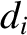the collisionless ion skin-depth at the rational surface [see Equation (4.24)]. Moreover,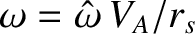is the rotation frequency of the tearing mode in the laboratory frame,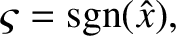(8.7)

and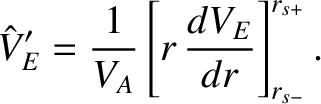(8.8)

The parameter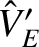is introduced into the analysis in order to take into account the fact that the E-cross-B velocity profile in the outer region develops a gradient discontinuity at the rational surface in response to the localized electromagnetic torque that emerges at the surface. Note, finally, that in neglecting any dependance of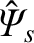on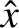in Equation (8.2) we are making use of the so-called constant-approximation [7,8], which is valid as long as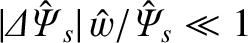, where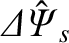is defined in Equations (3.73) and (3.183) [2,4].